# AA4 – Energy Transition

## Decision-Making for Energy Network Dynamics

Falk Hante, Michael Hintermüller, Sebastian Pokutta

Project Members

Paulina Bock de Barillas

Project Duration

01.06.2021 − 31.05.2024

Located at

HU Berlin

### Topic and Background of the Project

The transition to sustainable energy networks provides complexities arising from the integration of rather unsteady renewables (figure below). The unsteady network dynamics cause operational challenges for network providers. Mathematical control theory that includes modeling, simulation and optimization can support dispatching processes, but is facing challenges of coupling large scale dynamics from physical models such as partial differential equations (PDE) with discrete decision models such as 0-1 switching for connecting and disconnecting capacitors. These problems are to be solved fast and repeatedly while gathering data to adapt. The research agenda of this project tackles fundamental questions concerning control and optimization in context of this application.

### Basic model of the power network

Our basis is the model used in , where the power network is modeled as a graph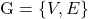with the set of all nodes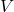, which may describe interlinked power stations, generators, consumers (power demand), transformers (that boost or lower voltage) and the set of all edges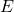which are the transmission lines between them. A transmission line is mathematically described by the telegraph equations, a coupled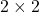system of equations that predict the voltage and current distributions on this single electrical transmission line with space coordinate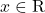and time coordinate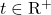.

(1)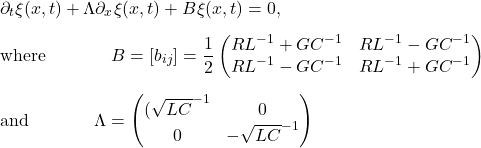The so called characteristic variables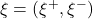, with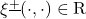represent right traveling components (i.e.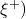and left traveling components (i.e.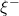). Inductance, capacity, resistance and the admittance per unit length of the conductor are represented by the constants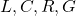. The voltage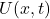and the current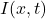can be computed out of the characteristic variables.
These equations are defined on the transmission lines (edges), we parametrize an edgeby the interval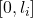and couple them at the nodes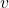by Kirchhoff’s conditions.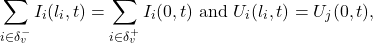for all incoming edges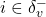and outgoing edges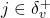at node v. In words, incoming power at a node equals power that leaves the node at the same time and all potentials (voltage) connected at a node must be equal.

The well known alternating current optimal power flow equations (ACOPF) are related to this model in the sense that any solution to the power flow model yields a solution to the telegraph equations with sinusodial boundary conditions.

### Relaxation

In one direction of our research we consider a mixed integer optimal control problem (MILP), with binary constraints on the control for turning generators on and off. Relaxing these constraints in the sense, that also rational solutions in the interval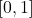are allowed, allows us to realize the minimization via first order optimality conditions. In our research we want to include an exact relaxation (see ), which means that there is no relaxation gap, i.e. the solution of the relaxed problem is the same as the solution of the original, non relaxed problem. The main advantage of this is that we can work with the relaxed problem, which is computationally much easier, and we can directly transfer the results to the original problem.
We want to emphasize, that this is contrary to the Sum Up Rounding technique for MIP(see , , ). It is a strategy to round the solution of the relaxed problem to obtain an integer solution and delivers an upper bound for the integral over the difference of the relaxed and rounded control (see fig. 2 for an example). This bound depends on the discretization of the control, meaning that the finer the grid, the smaller bound gets and the optimal objective function value is reached up to an arbitrarily small-gap.

### Combinatiorial Constraints

Usually we want to avoid very short successive changes of the integer control. This requirement can be expressed by additional constraints on the integer control. Such combinatorial (or dwell time) contraints describe the necessity of activation/deactivation of an integer control for at least a given minimal duration.
A discretization of such problems leads to mixed integer nonlinear problems, that become computationally intractable when the discretization stepsize tends to zero.
We consider another solution approach based on the idea of alternating direction methods, with a clear separation of the combinatorial aspects from the constinuous control aspects. In one direction we use partial outer convexification (POC) and combinatiorial integral approximation (CIAP) and in the other direction we solve an mixed integer problem. These two directions are weakly coupled by a penalty parameter. Based on the exactness of the penalty parameter, we can derive a convergent result for this method. (See  in publications).

Related Publications

 A. Bärmann, A. Heidt, A. Martin, S. Pokutta and C. Thurner: Polyhedral Approximation of Ellipsoidal Uncertainty Sets via Extended Formulations – a computational case study. Computational Management Science, Vol. 13, Nr. 2, 2016.
 G. Braun, A. Roy and S. Pokutta. Stronger Reductions for Extended Formulations. arXiv preprint arXiv:1512.04932 (2018).
 M. Burger, Y. Dong, and M. Hintermüller: Exact relaxation for classes of minimization problems with binary constraints, arXiv preprint arXiv:1210.7507 (2012).
 S. Fiorini, S. Massar, S. Pokutta, H.R. Tiwary, and R. de Wolf: Linear vs. Semidefinite Extended Formulations: Exponential Separation and Strong Lower Bounds. Proceedings of STOC 2012. arXiv:1111.0837 (2012).

 Hante, F.M. et al. Challenges in optimal control problems for gas and fluid flow in networks of pipes and canals: From modeling to industrial applications. In: Industrial Mathematics and Complex Systems, P. Manchanda, R. Lozi, A.H. Siddiqi (Eds.), Springer Singapore, pp. 77–122, 2017.
 F.M. Hante, and S. Sager: Relaxation methods for mixed-integer optimal control of partial differential equations, Computational Optimization and Applications, 2013.
 Hante, F.M.: Relaxation methods for hyperbolic PDE mixed-integer optimal control problems. Optimal Control Applications and Methods. Vol. 38, Nr. 6, pp. 1103–1110, 2017.
 F.M. Hante: Mixed-Integer Optimal Control for PDEs: Relaxation via Differential Inclusions and Applications to Gas Network Optimization. In: Industrial and Applied Mathematics, P. Manchanda, R. Lozi, A.H. Siddiqi (Eds.), pp. 157–171 Springer Singapore, 2020.
 M. Hintermüller, K. Ito, and K. Kunisch: The primal-dual active set strategy as a semismooth Newton method. SIAM Journal on Optimization, Vol 13, Nr. 3, 2002.

 S. Göttlich, M. Herty, and P. Schillen. Electric transmission lines: control and
numerical discretization. Optimal Control Appl. Methods, 37(5):980–995, 2016.

 M. Burger, Y. Dong, and M. Hintermüller. Exact Relaxation for Classes of Mini-
mization Problems with Binary Constraints, Oct. 2012.
 P. Manns and C. Kirches. Multidimensional sum-up rounding for elliptic control
systems. SIAM Journal on Numerical Analysis, 58(6):3427–3447, 2020.
 S. Sager. Numerical methods for mixed–integer optimal control problems. Der andere Verlag, Tönning, Lübeck, Marburg, 2005. ISBN 3-89959-416-9.
 S. Sager, H. G. Bock, and M. Diehl. Solving Mixed–Integer Control
Problems By Sum Up Rounding With Guaranteed Integer Gap.(2007)

Completed Work

Publications

 S. Göttlich, F.M. Hante, A. Potschka, and L. Schewe: Penalty alternating direction methods for mixed-integer optimal control with combinatorial constraints. Mathematical Programming, Vol. 188, pp. 599–619 (2021).

 F.M. Hante: Relaxation methods for optimal switching control of PDE-dynamical systems. Handbook of Numerical Analysis, Numerical Control: Part B, Enrique Zuazua and E. Trelat (Eds.), Volume 24, 2023, Pages 61-76 (2022).
 F.M. Hante, R. Krug, M. Schmidt: Time-Domain Decomposition for Mixed-Integer Optimal Control Problems. Applied Mathematics and Optimization, Volume 87, Number 36 (2023).
 F.M. Hante, M. Schmidt: Gas Transport Network Optimization: Mixed-Integer Nonlinear Models. To appear in Encyclopedia of Optimization, 3rd ed. (Panos M. Pardalos and Oleg Prokopyev, Eds.), Springer Nature, 2024.

 F.M. Hante, M. Schmidt: Gas Transport Network Optimization: PDE-Constrained Models. To appear in Encyclopedia of Optimization, 3rd ed. (Panos M. Pardalos and Oleg Prokopyev, Eds.), Springer Nature, 2024.

Master thesis

 Till Schäfer, Regularization of Mixed-Integer Control Approximations based on Relaxation and Optimization, 24.03.2023

 Xinglei Liu, An Augmented Lagrangian Decomposition Approach for Power Grid Optimization, 03.07.2023